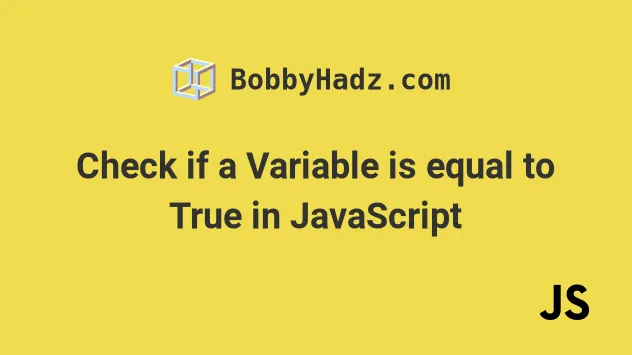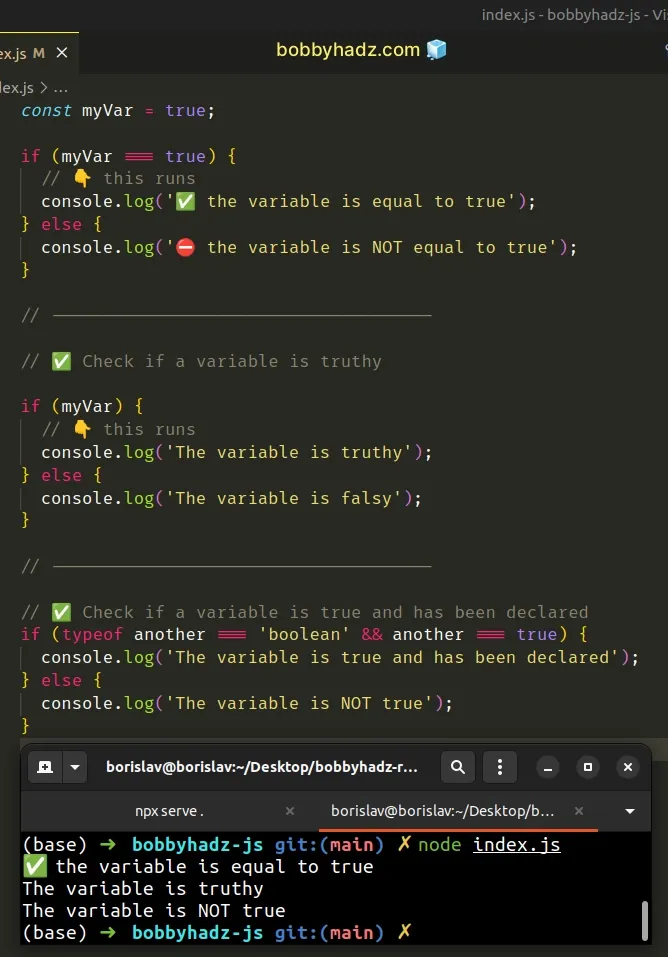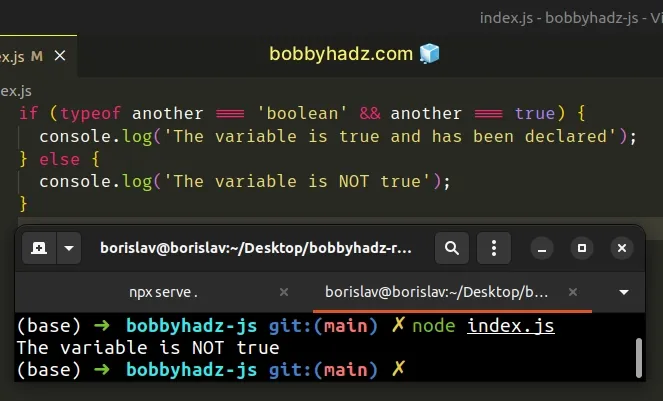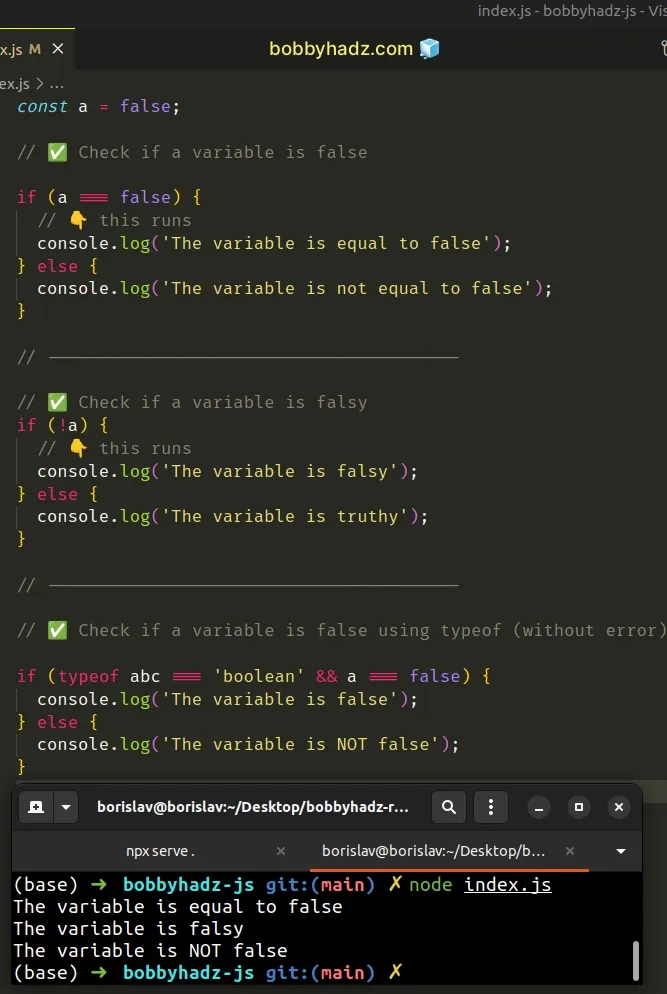# Check if a Variable is equal to True in JavaScriptLast updated: Dec 27, 2022
5 min## #Check if a Variable is equal to True in JavaScript

Use the strict equality (===) operator to check if a variable is equal to `true`.

The strict equality operator will return `true` if the variable is equal to `true`, otherwise, it will return `false`.

index.js
```Copied!```const myVar = true;

if (myVar === true) {
// 👇️ this runs
console.log('✅ the variable is equal to true');
} else {
console.log('⛔️ the variable is NOT equal to true');
}

// -------------------------------------

// ✅ Check if a variable is truthy

if (myVar) {
// 👇️ this runs
console.log('The variable is truthy');
} else {
console.log('The variable is falsy');
}

// -------------------------------------

// ✅ Check if a variable is true and has been declared
if (typeof another === 'boolean' && another === true) {
console.log('The variable is true and has been declared');
} else {
console.log('The variable is NOT true');
}
``````If you need to check if a variable is `False`, click on the following subheading:

Check if a Variable is False in JavaScript

We used the strict equality (===) operator to check if the value stored in the `myVar` variable is equal to `true`.

The operator returns a boolean result:

• `true` if the values are equal
• `false` if the values are not equal
The strict equality (===) operator considers two values of different types to be different, as opposed to the loose equality (==) operator.

This means that if we compare `true` with any other type, the strict equality operator (===) would return `false`.

index.js
```Copied!```console.log(true === true); // 👉️ true
console.log(true === 'true'); // 👉️ false
console.log(true === 1); // 👉️ false
``````

A very common mistake is to check if a value is truthy, instead of checking if it is equal to `true`. Here's an example.

index.js
```Copied!```const myVar = true;

if (myVar) {
console.log(`🚨 a is NOT
false, 0, empty string, null, undefined, NaN`);
} else {
console.log(`⛔️️ a is ONE OF
false, 0, empty string, null, undefined, NaN`);
}
``````

The `if` statement checks if the `myVar` variable is truthy. Truthy are all values that are not falsy.

The falsy values in JavaScript are: `false`, `0`, `""`, `null`, `undefined`, `NaN`.

This means that, for the `if` block to run, the value stored in the `myVar` variable can be anything other than the aforementioned `6` falsy values. It doesn't necessarily have to be equal to `true`.

Things can go wrong when you use this approach to check if a variable is equal to `true`. Here's an example.

index.js
```Copied!```const myVar = [];

if (myVar) {
console.log('✅ this runs');
} else {
console.log("⛔️ this doesn't run");
}
``````

The empty array is considered a truthy value, so the `if` block runs.

Checking if a variable stores a truthy value is not necessarily bad, you just have to be aware of the difference between `true` and truthy.

It's always good to be explicit when checking if a variable is equal to a specific value.

index.js
```Copied!```const myVar = [];

if (myVar === true) {
console.log("⛔️ this doesn't run");
} else {
console.log('✅ this runs');
}
``````

Being explicit when comparing values makes your code more readable and direct.

## #Check if a variable is equal to `true` using `typeof`

Alternatively, you can use the `typeof` operator.

The `typeof` operator will check if the variable is equal to `true` and won't cause an error even if the variable has not been declared in the scope.

index.js
```Copied!```if (typeof another === 'boolean' && another === true) {
console.log('The variable is true and has been declared');
} else {
console.log('The variable is NOT true');
}
``````We didn't declare the `another` variable but running the code sample doesn't cause an error because we used the `typeof` operator.

The `typeof` operator returns a string that indicates the type of a value.

We used the logical AND (&&) operator, so for the `if` block to run both conditions have to be met.

The variable has to have a type of `boolean` and it has to be equal to the value `true`.

## #Check if a Variable is False in JavaScript

Use the strict equality (===) operator to check if a variable is equal to `false`.

The strict equality (===) operator will return `true` if the variable is equal to `false`, otherwise, it will return `false`.

index.js
```Copied!```const a = false;

// ✅ Check if a variable is false

if (a === false) {
// 👇️ this runs
console.log('The variable is equal to false');
} else {
console.log('The variable is not equal to false');
}

// ----------------------------------------

// ✅ Check if a variable is falsy
if (!a) {
// 👇️ this runs
console.log('The variable is falsy');
} else {
console.log('The variable is truthy');
}

// ----------------------------------------

// ✅ Check if a variable is false using typeof (without error)

if (typeof abc === 'boolean' && a === false) {
console.log('The variable is false');
} else {
console.log('The variable is NOT false');
}
``````We used the strict equality (===) operator to check if a variable stores a `false` value.

The operator returns a boolean value:

• `true` if the values are equal
• `false` if the values are not equal
The strict equality (===) operator considers two values of different types to be different, as opposed to the loose equality (==) operator.

This means that if we compare `false` with any other type, the strict equality operator (===) would return `false`.

index.js
```Copied!```console.log(false === false); // 👉️ true
console.log(false === 'false'); // 👉️ false
console.log(false === 0); // 👉️ false
``````

A very common mistake is to check if a value is falsy, instead of checking if it is equal to `false`.

Here's an example.

index.js
```Copied!```const a = false;

if (!a) {
console.log(`⛔️️ a is ONE OF
false, 0, empty string, null, undefined, NaN`);
} else {
console.log(`🚨 a is NOT
false, 0, empty string, null, undefined, NaN`);
}
``````

We used the logical NOT (!) operator to flip the value of the variable.

Our `if` statement checks if the variable is falsy.

The falsy values in JavaScript are: `false`, `0`, `""` (empty string), `null`, `undefined`, `NaN` (not a number).

This means that for the `if` block to run, the variable could be any of the aforementioned `6` falsy values and not necessarily `false`.

Here is an example of how this could cause issues.

index.js
```Copied!```const a = 0;

if (!a) {
console.log('✅ this runs');
} else {
console.log("⛔️ this doesn't run");
}
``````

The `if` block runs because the variable is set to `0` which is a falsy value.

This is confusing to the person reading the code because the `if` block would run if the variable is set to any of the `6` falsy values.

Instead, it's better to be more explicit.

index.js
```Copied!```const a = 0;

if (a !== 0) {
console.log("⛔️ this doesn't run");
} else {
console.log('✅ this runs');
}
``````

Being explicit when comparing values saves yourself and the developers reading your code time.

## #Check if a variable is equal to `false` using `typeof`

Alternatively, you can use the `typeof` operator.

The `typeof` operator will check if the variable is equal to `false` without throwing an error if the variable has not been declared.

index.js
```Copied!```if (typeof abc === 'boolean' && abc === false) {
console.log('The variable is false');
} else {
// 👇️ this runs
console.log('The variable is NOT false');
}
``````

Notice that the `abc` variable has not been declared, but no error occurs when running the code sample.

The `typeof` operator returns a string that indicates the type of a value.

The operator won't cause an error even if the variable you are checking for has not been declared.

We used the logical AND (&&) operator to chain multiple conditions.

The `if` block will run only if both conditions are met.